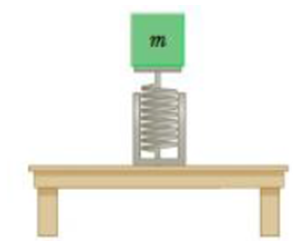Chapter 13, Problem 2P

Chapter
Section
Textbook Problem

A spring oriented vertically is attached to a hard horizontal surface as in Figure P13.2. The spring has a force constant of 1.46 kN/m. How much is the spring compressed when a object of mass m = 2.30 kg is placed on top of the spring and the system is at rest?Figure P13.2

To determine
To determine the compression of the spring.

Explanation

Given Info: The force constant of the spring is 1.46kNm-1 . The mass of the spring m is 2.30kg .

Since the mass m is placed on top of the spring, acceleration due to gravity will work on it. So, acceleration a is equal to g.

From Hooke’s law, the formula for the force is,

F=kx (I)

• F is the force
• k is force constant of the spring
• x is the displacement of the spring

The force is equal to the force of gravity.

F=mg (II)

• m is the mass of the object
• g is the acceleration due to gravity

From Equations (I) and (II),

kx=mgx=mgk

Substitute 1.46kNm-1 for k , 2

Still sussing out bartleby?

Check out a sample textbook solution.

See a sample solution

The Solution to Your Study Problems

Bartleby provides explanations to thousands of textbook problems written by our experts, many with advanced degrees!

Get Started